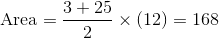ISEE Lower Level Math : Trapezoids

Example Questions

Example Question #11 : Trapezoids

Find the area of the trapezoid.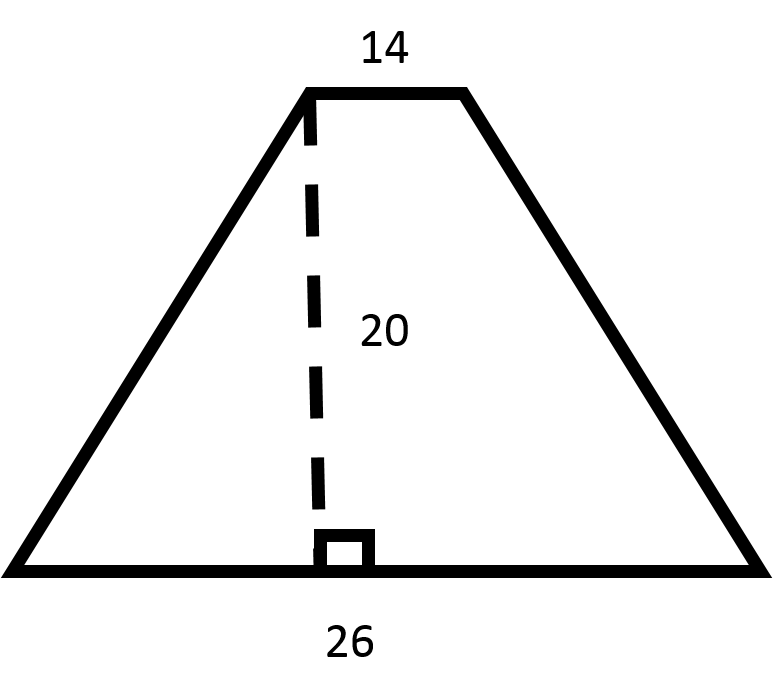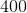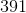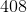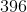Explanation:

Recall the formula for finding the area of a trapezoid: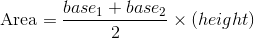Now, plug in the values for the bases and the height to find the area.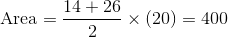Example Question #12 : Trapezoids

Find the area of the trapezoid.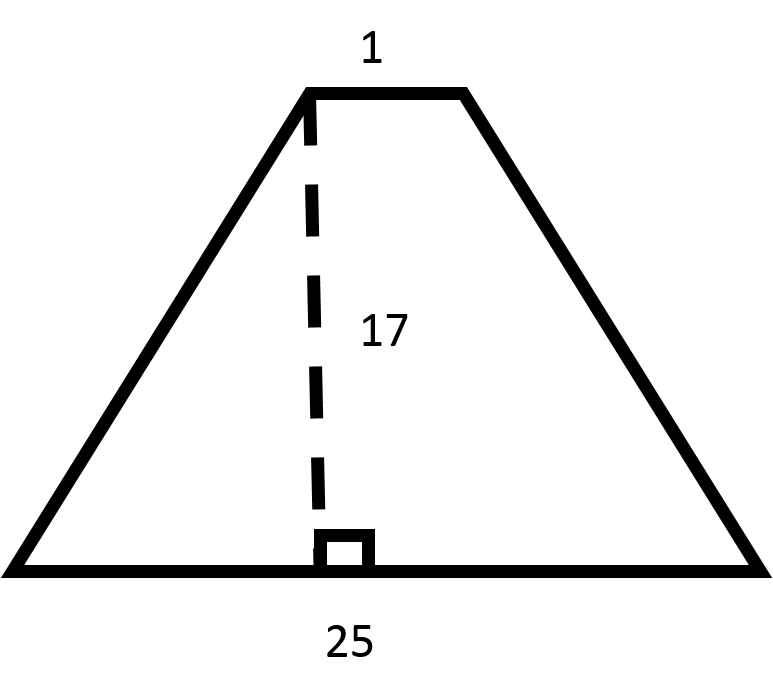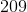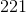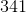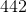Explanation:

Recall the formula for finding the area of a trapezoid:Now, plug in the values for the bases and the height to find the area.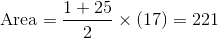Example Question #13 : Trapezoids

Find the area of the trapezoid.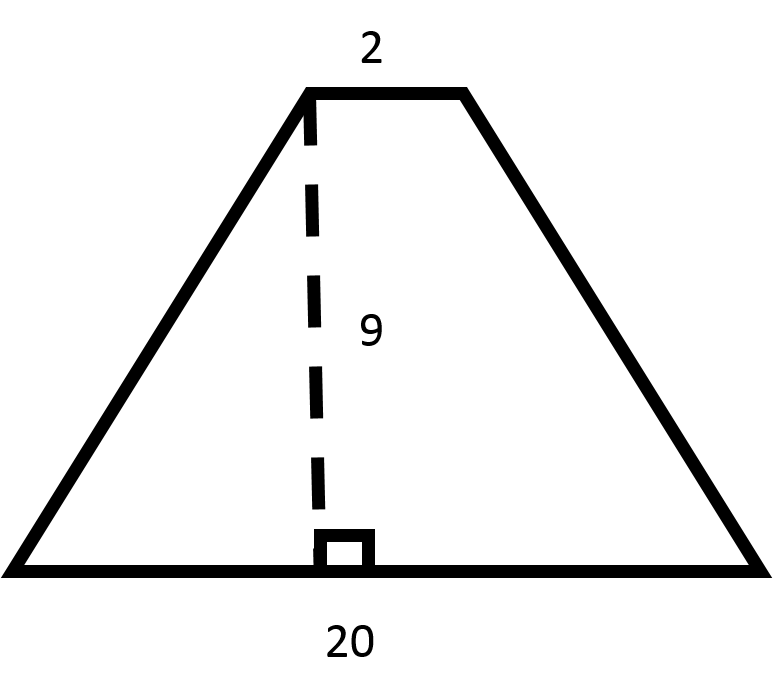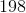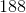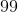Explanation:

Recall the formula for finding the area of a trapezoid:Now, plug in the values for the bases and the height to find the area.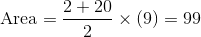Example Question #14 : Trapezoids

Find the area of the trapezoid.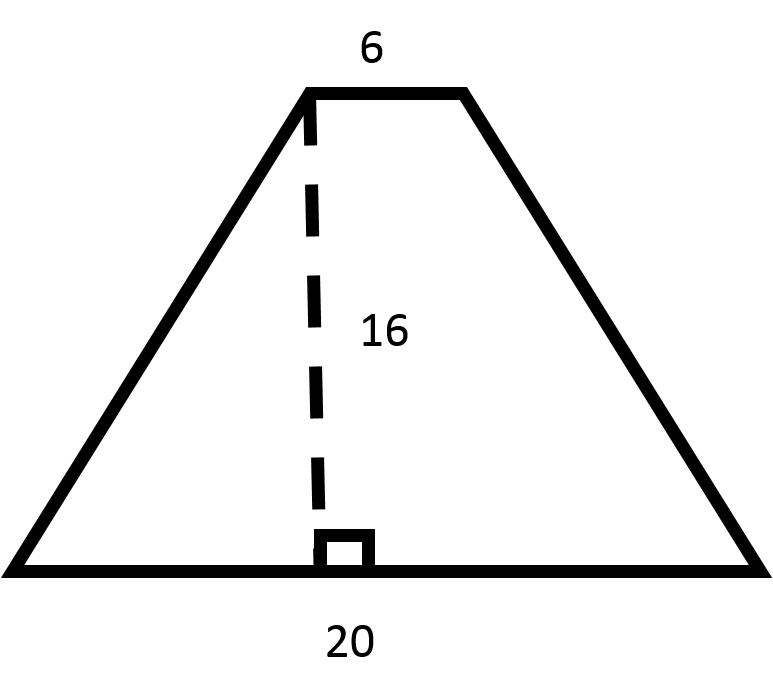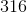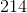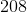Explanation:

Recall the formula for finding the area of a trapezoid:Now, plug in the values for the bases and the height to find the area.Example Question #15 : Trapezoids

Find the area of the trapezoid.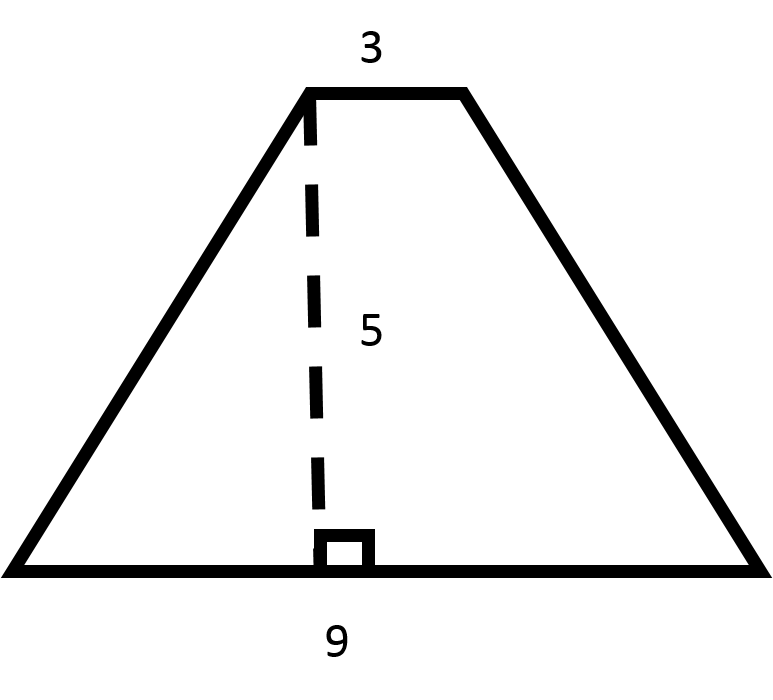Explanation:

Recall the formula for finding the area of a trapezoid:Now, plug in the values for the bases and the height to find the area.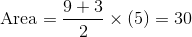Example Question #16 : Trapezoids

Find the area of the trapezoid.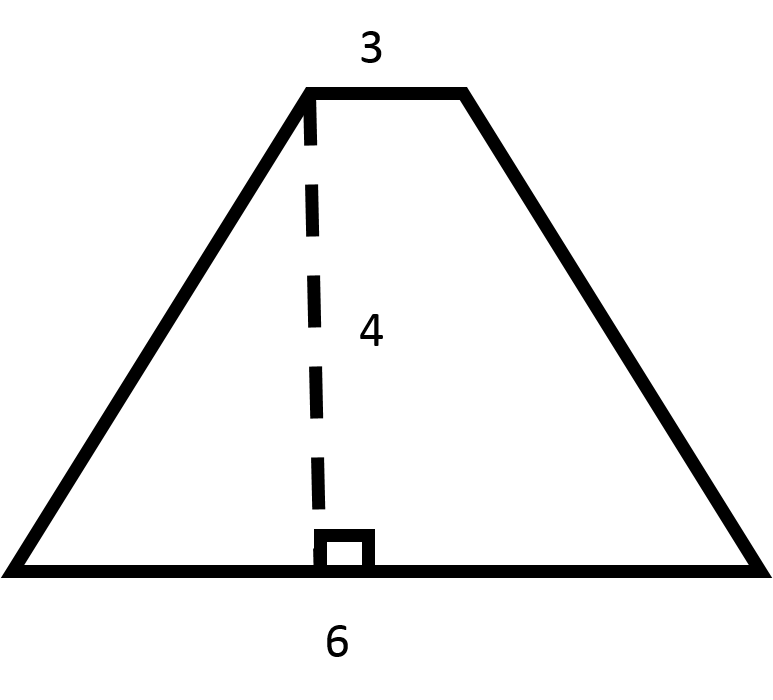Explanation:

Recall the formula for finding the area of a trapezoid:Now, plug in the values for the bases and the height to find the area.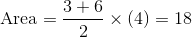Example Question #17 : Trapezoids

Find the area of the trapezoid.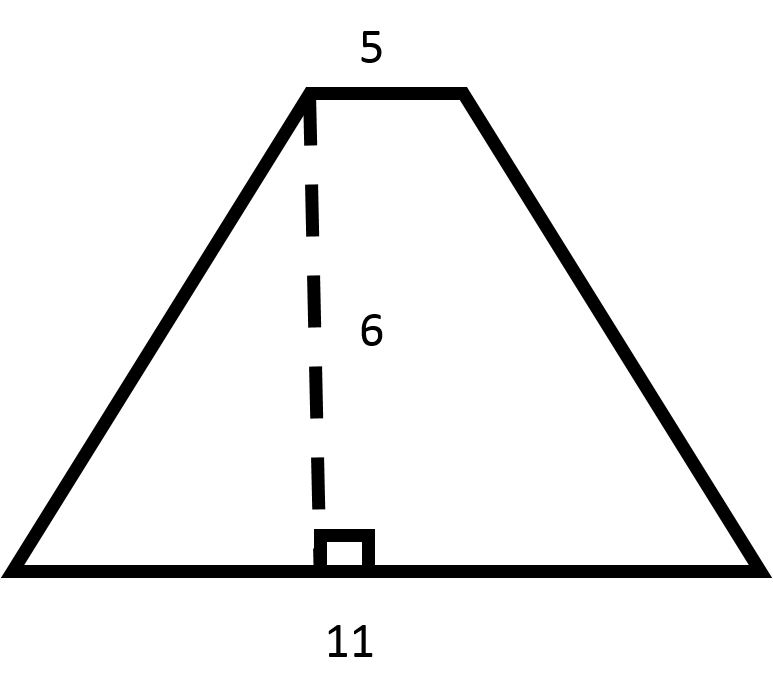Explanation:

Recall the formula for finding the area of a trapezoid:Now, plug in the values for the bases and the height to find the area.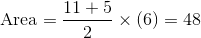Example Question #18 : Trapezoids

Find the area of the trapezoid.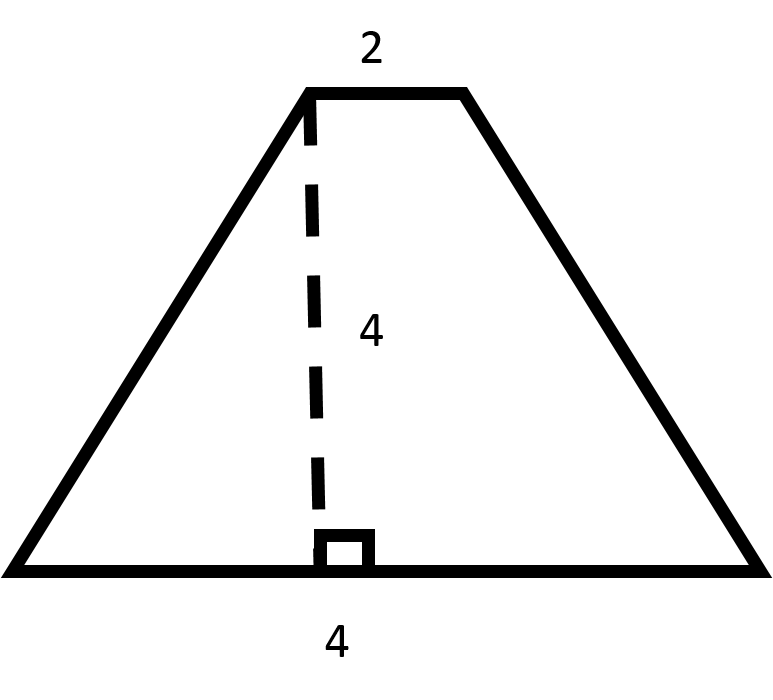Explanation:

Recall the formula for finding the area of a trapezoid:Now, plug in the values for the bases and the height to find the area.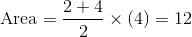Example Question #19 : Trapezoids

Find the area of the trapezoid.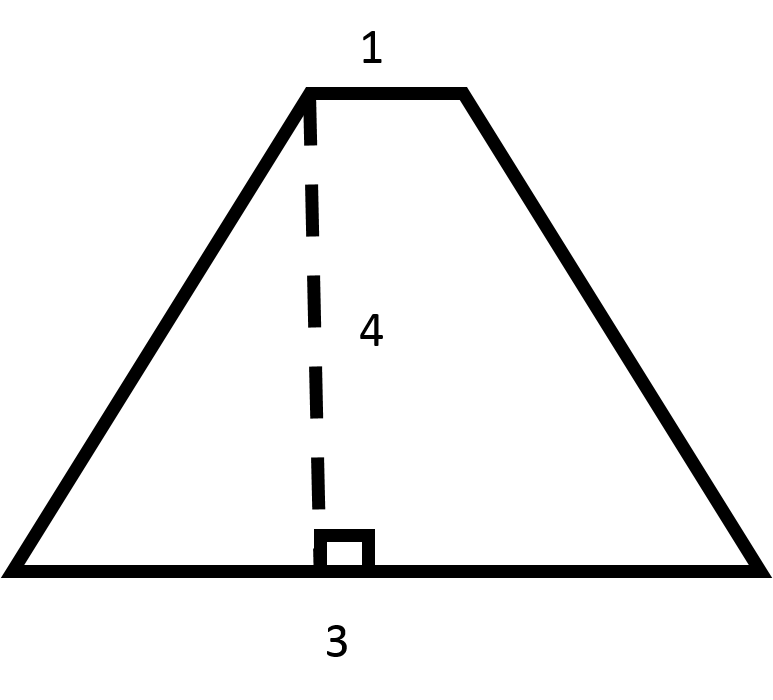Explanation:

Recall the formula for finding the area of a trapezoid:Now, plug in the values for the bases and the height to find the area.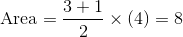Find the area of the trapezoid.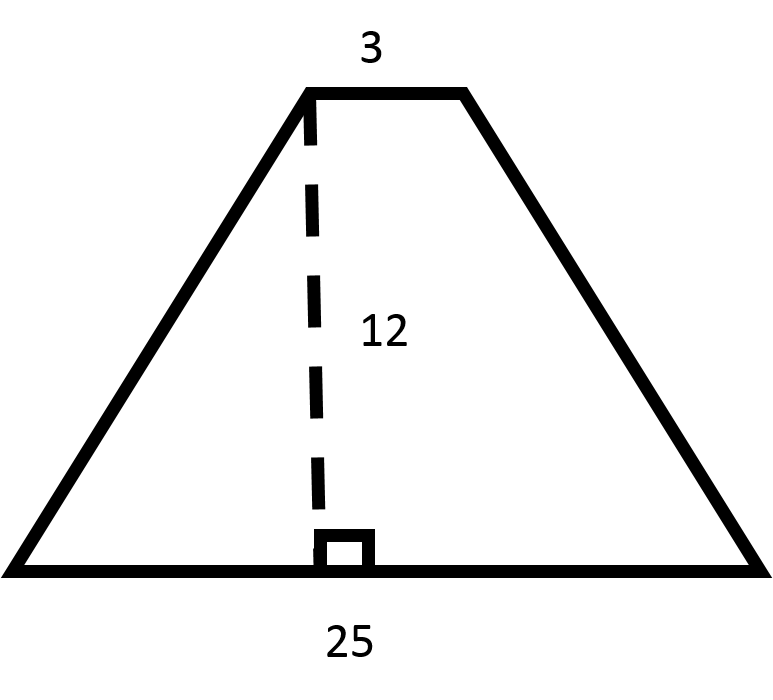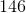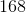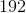Explanation:

Recall the formula for finding the area of a trapezoid:Now, plug in the values for the bases and the height to find the area.